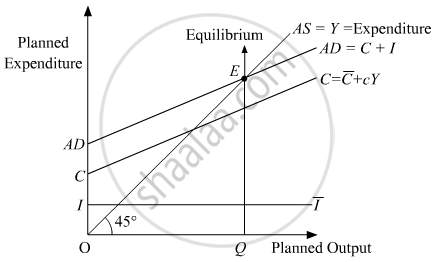Advertisement Remove all ads

# Answer the Following Question. What is Meant by the "Effective Demand Principle" in the Keynesian Theory of Employment? Discuss Using a Schedule Or a Diagram. - Economics

Advertisement Remove all ads
Advertisement Remove all ads
Advertisement Remove all ads
Answer in Brief

Answer the following question.
What is meant by the "Effective Demand Principle" in the Keynesian theory of employment? Discuss using a schedule or a diagram.

Advertisement Remove all ads

#### Solution

Effective Demand Principle refers to a situation in which the equilibrium output is determined solely by the level of aggregate demand. If there exists any inequality between AD and AS, then equilibrium output is influenced only by AD and AS has to be adjusted in such a way that it equals AD.

According to the AD-AS approach, the equilibrium level of income is determined at that point, where Aggregate Demand (AD) is equal to Aggregate Supply (AS). It should be noted that here AD refers to the planned/desired level of expenditure in the economy during an accounting year. Similarly, it refers to the planned/desired level of output in an economy during an accounting year.In the diagram, the consumption curve is depicted by C and the investment curve is depicted by the horizontal straight line parallel to the output/income axis. Summing-up the investment curve and consumption curve we get the Aggregate Demand curve represented by AD = C + I. The Aggregate Supply curve is represented by the 45° line. Throughout this line, the planned expenditure is equal to the planned output. That is AS = Y = Expenditure. The implication of 45° line is that in case of any disequilibrium, AS will be adjusted in a way to equate AD in order to restore equilibrium back. That is in other words, in case of any inequality between AD and AS, equilibrium output will be determined by AD. The point E is the equilibrium point, where the planned level of expenditure (AD) is equal to the planned level of output (AS). In other words, this suggests that there is no undesired inventory accumulation. The equilibrium level of output is OQ, which is also known as the 'effective demand'.

Concept: Concept of Aggregate Demand and Aggregate Supply
Is there an error in this question or solution?

#### APPEARS IN

Advertisement Remove all ads
Share
Notifications

View all notifications

Forgot password?